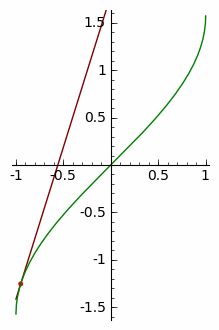jsMath

# Derivatives of inverse functions

 ```Suppose f is a one-to-one function. For every point P1=(a,b)on the graph of y=f(x), there is a corresponding point P2=(b,a) on thegraph of y=f−1(x) . The points P1 and P2 are reflections of each otherover the line y=x. In the illustration below, a=1.2 and b=3.32.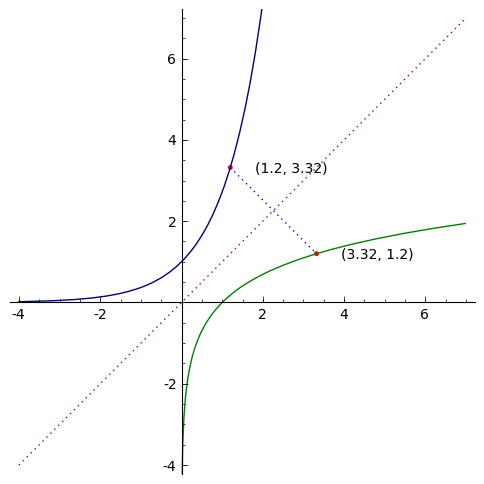Now, suppose we have a line tangent to y=f(x) at the point (a,b). Theslope m of this line will be m=f′(a). Since the graph of y=f−1(x)  isthe reflection of the graph of y=f(x), the line tangent to y=f−1(x) at (b,a) will have a slope of 1/m , the reciprocal of m.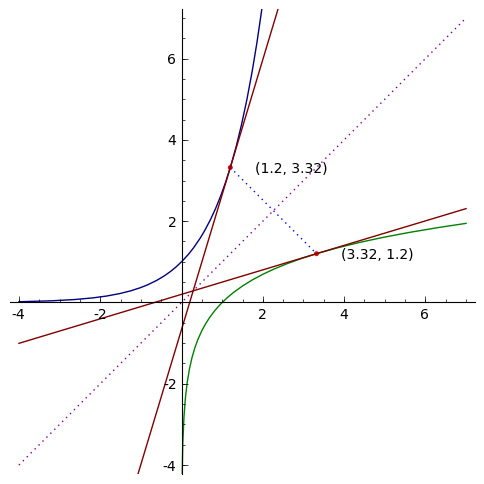Here is an animation showing the two tangent lines at various values of a:```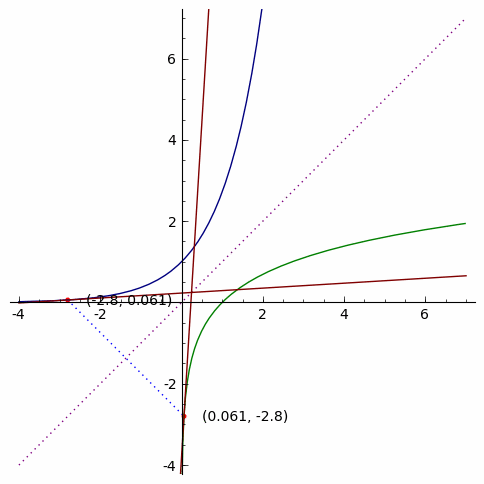```Here is the graph of y=sinx, with domain restricted to [−π/2,π/2] ,and the inverse function y=arcsinx.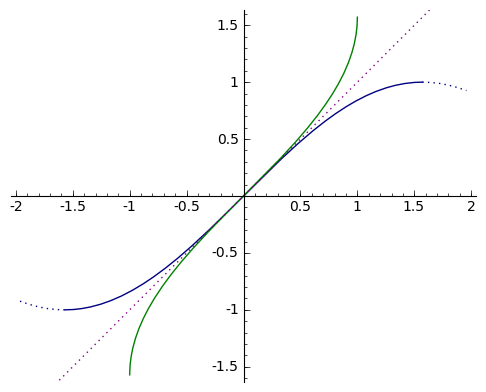Here in an animation showing the lines tangent to y=sinx at (a,sina) and y=arcsinx at (sina,a), for various values of a:```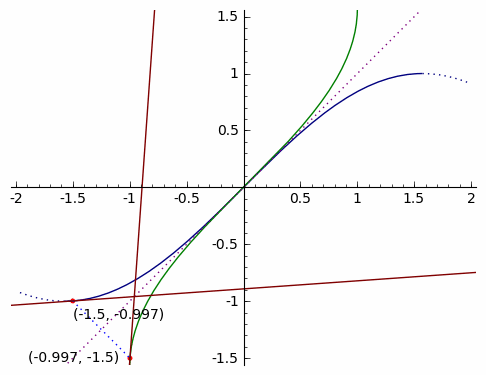```Here is a plot of f(x)=arcsinx, an animated tangent line,and the derivative f′(x)=1√1−x2.(The derivative may appear first.)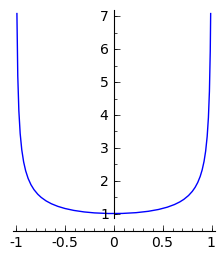```